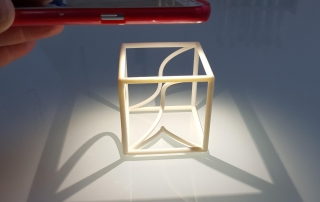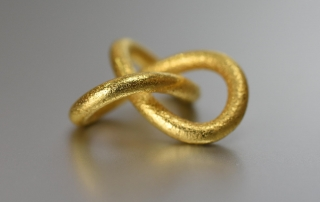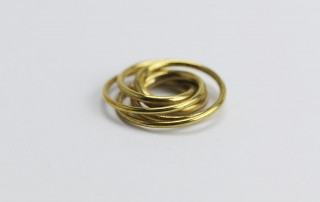•Space curve in a cube with projections (1b)
Gallery

Space curve in a cube with projections (1b)

This space curve in a cube with projections (1b) is a math classic. Use a torch to compare the projections of the space curve (in the center) on a plane with the 3d-printed ones.

Space curve in a cube without projections (1a)

This space curve in a cube is a math classic. To view its projections on a plane, just take a torch (or your cell phone lamp).

•A trefoil knot pendant
Gallery

A trefoil knot pendant

A trefoil knot is the simplest non-trivial mathematical knot. It has been known for thousands of years.

•Speed Curve pendant
Gallery

Speed Curve pendant

At first sight, our speed curve pendant might seem to consist of some arbitrary wire. But this is not true at all. Almost every detail is defined by mathematical formulas.